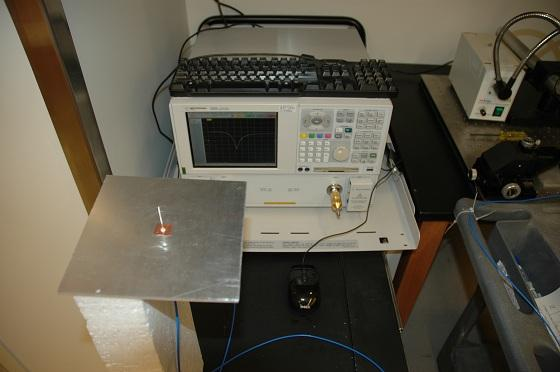# Monopole Measurement Comparison

This example compares the impedance of a monopole analyzed in Antenna Toolbox™ with the measured results. The corresponding antenna was fabricated and measured at the Center for Metamaterials and Integrated Plasmonics (CMIP), Duke University. The monopole is designed for an operating frequency of 2.5 GHz.

### Create, Modify and View Monopole

Create the default monopole antenna geometry. Then, modify the height and the width of the monopole, and dimensions of the ground plane to be in agreement with the hardware prototype. Since the monopole is located at the center of the ground plane, the FeedOffset property of the antenna is not modified.

```mp = monopole; mp.Height = 28.5e-3; mp.Width = 2.54e-3; mp.GroundPlaneLength = 0.1; mp.GroundPlaneWidth = 0.1; figure; show(mp)```### Plot Impedance and Return Loss

Define the frequency band for the analysis. The lower band frequency is 500 MHz and the upper band frequency is 5 GHz. Since the reference impedance is not specified as one of the arguments when calculating the return loss, the default value of 50 $\Omega$ is used.

```freq = 0.5e9:50e6:5e9; RL = returnLoss(mp,freq); [~,ind1] = max(RL); figure; impedance(mp,freq); marker1 = linspace(-500,500,21); hold on plot(freq(ind1).*ones(1,21)./1e9,marker1,'m-.','LineWidth',2) textInfo = [ ' \leftarrow' num2str(freq(ind1)/1e9) 'GHz']; text(freq(ind1-1)/1e9,300,textInfo,'FontSize',11) hold off``````figure returnLoss(mp,freq); marker2 = linspace(0,30,21); hold on plot(freq(ind1).*ones(1,21)./1e9,marker2,'m-.','LineWidth',2) hold off```### Comparison with Measurement - Reflection Coefficient

Measurements of the fabricated monopole are taken over the same frequency band. The measured data is the reflection coefficient (${S}_{11}$) of the monopole in decibels. To compare with measurements, plot the numerical reflection coefficient, which is equal to the negative return loss.

```load('monopole_measured.mat'); figure; plot(freq/1e9,-mp.returnLoss(freq),'b','linewidth',3); hold on; plot(f/1e9,S11dB,'r','linewidth',3); hold off; grid on legend('Analysis','Measurement','Location','Best'); xlabel('Frequency (GHz)'); ylabel('S_1_1 (dB)'); title('Monopole Antenna');```The figure indicates a good agreement between theory and measurements. Typically, a criterion such as ${S}_{11}<-10dB$ is used for describing a good impedance match. The analysis and measurement confirm that the monopole satisfies the criterion in a band centered about 2.5 GHz. The fabricated monopole and the measurement setup are shown below.Fabricated monopole, and measurement setup (with permission from CMIP, Duke University)